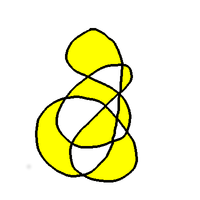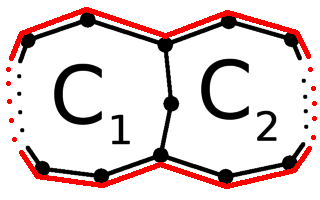The Doodle TheoremSubscribe!My latest posts can be found here:
Previous blog posts:
Additionally, some earlier writings:
 This page has been Tagged As Maths.

The Doodle Theorem

The Doodle Theorem says:

 Any map drawn with a single pen stroke that returns to its starting point can be two-coloured.

Here's one proof.Take a planar graph that's been drawn with a single pen stroke that returns to its starting point.

• As we draw the graph, for every vertex we must arrive, then depart.

• Except for the vertex where we start and end, but we can think of the final arrival as matched with the initial departure.

• As a result, every vertex must have an even number of edges.

• In the dual, a circuit around a vertex of the original will have an even number of edges,

• ... because edges in the dual cross edges in the original.• Consider two circuits that share edges but do not cross. When combined into a single cycle, the length of the resulting cycle is the sum of the two lengths, minus twice the number of edges in the shared section.

• By combining the individual cycles in the dual around vertices of the original map, we can now see that every cycle in the dual is of even length.

Now we use the theorem that says:

• A graph is bipartite if and only if every circuit is even.

We'll come back to that in a minute.

 An Euler Cycle is a tour of all the edges of a graph, visiting vertices as often as necessary, but traversing every edge exactly once, and returning to our starting position.
So given all that, we now have that the dual is bipartite, and that means both it and the original map can be bi-coloured!

So we're done - a planar graph with an Euler Cycle (see side box) can be bi-coloured.

Well, nearly done. We still have to show that last step.

 Theorem: A graph is bipartite if and only if Every circuit is even. Proof: It's enough to prove this for each component, so we may assume that the graph is connected. Pick any vertex $a$ and put it in $A.$ Repeatedly pick a vertex $v$ in $A$ and put its neighbours in $B,$ or pick a vertex in $B$ and put its neighbours in $A.$ If a vertex is put both in $A$ and in $B$ (for the first time), that gives us an odd cycle. If this never happens, then the sets $A$ and $B$ form a partition of the vertices of the graph into two independent sets; in other words, it is a bipartite graph. And we really are done.

 <<<< Prev <<<< Be Careful What You Say : >>>> Next >>>> Graph Three Colouring ...

 You should follow me on twitterI've decided no longer to include comments directly via the Disqus (or any other) system. Instead, I'd be more than delighted to get emails from people who wish to make comments or engage in discussion. Comments will then be integrated into the page as and when they are appropriate.

If the number of emails/comments gets too large to handle then I might return to a semi-automated system. That's looking increasingly unlikely.

ContentsSuggest a change ( <-- What does this mean?) / Send me email